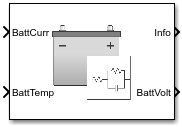# Equivalent Circuit Battery

Resistor-capacitor (RC) circuit battery

• Library:
• Powertrain Blockset / Energy Storage and Auxiliary Drive / Network Battery

•## Description

The Equivalent Circuit Battery block implements a resistor-capacitor (RC) circuit battery that you can parameterize using equivalent circuit modeling (ECM). To simulate the state-of-charge (SOC) and terminal voltage, the block uses load current and internal core temperature.

The Equivalent Circuit Battery block calculates the combined voltage of the network battery using parameter lookup tables. The tables are functions of the SOC and battery temperature. You can use the Estimation Equivalent Circuit Battery block to help create the lookup tables.

Specifically, the Equivalent Circuit Battery block implements these parameters as lookup tables that are functions of the SOC and battery temperature:

• Series resistance, Ro=ƒ(SOC,T)

• Battery open-circuit voltage, Em=ƒ(SOC,T)

• Battery capacity, Cbatt=ƒ(T)

• Network resistance, Rn=ƒ(SOC,T)

• Network capacitance, Cn=ƒ(SOC,T)

To calculate the combined voltage of the battery network, the block uses these equations.

`$\begin{array}{l}{V}_{T}={E}_{m}-{I}_{batt}{R}_{o}-\sum _{1}^{n}{V}_{n}\\ {V}_{n}=\underset{0}{\overset{t}{\int }}\left[\frac{{I}_{batt}}{{C}_{n}}-\frac{{V}_{n}}{{R}_{n}{C}_{n}}\right]dt\\ SOC=\frac{-1}{{C}_{batt}}\underset{0}{\overset{t}{\int }}{I}_{batt}dt\\ {I}_{batt}=\frac{{I}_{in}}{{N}_{p}}\\ {V}_{out}={N}_{s}{V}_{T}\\ {P}_{BattLoss}={I}_{batt}{}^{2}{R}_{0}+\sum _{1}^{n}\frac{{V}_{n}{}^{2}}{{R}_{n}}\\ L{d}_{AmpHr}=\underset{0}{\overset{t}{\int }}{I}_{batt}dt\end{array}$`

Positive current indicates battery discharge. Negative current indicates battery charge.

### Power Accounting

For the power accounting, the block implements these equations.

Bus Signal DescriptionEquations

`PwrInfo`

`PwrTrnsfrd` — Power transferred between blocks

• Positive signals indicate flow into block

• Negative signals indicate flow out of block

`PwrLdBatt`

Battery network power

`PwrNotTrnsfrd` — Power crossing the block boundary, but not transferred

• Positive signals indicate an input

• Negative signals indicate a loss

`PwrLossBatt`

Battery network power loss

${P}_{LossBatt}=-\left({I}_{batt}{}^{2}{R}_{0}+\sum _{1}^{n}\frac{{V}_{n}{}^{2}}{{R}_{n}}\right)$

`PwrStored` — Stored energy rate of change

• Positive signals indicate an increase

• Negative signals indicate a decrease

`PwrStoredBatt`

Battery network power stored

${P}_{StoredBatt}={P}_{Batt}+{P}_{LossBatt}$

The equations use these variables.

 SOC State-of-charge Em Battery open-circuit voltage Ibatt Per module battery current Iin Combined current flowing from the battery network Ro Series resistance Np Number parallel branches Np Number of RC pairs in series Vout, VT Combined voltage of the battery network Vn Voltage for `n`-th RC pair Rn Resistance for `n`-th RC pair Cn Capacitance for `n`-th RC pair Cbatt Battery capacity Pbatt Battery power PLossBatt Negative of battery network power loss PBattLoss Battery network power loss PStoredBatt Battery network power stored PLdBatt Battery network power T Battery temperature

## Ports

### Inputs

expand all

Rated battery capacity at the nominal temperature, Capbatt, in Ah.

#### Dependencies

To create this port, select ```External Input``` for the Initial battery capacity parameter.

Combined current flowing from the battery network, Iin, in A.

Battery temperature, T, in K.

### Output

expand all

Bus signal containing these block calculations.

SignalDescriptionVariableUnits

`BattCurr`

Combined current flowing from the battery network

Ibatt

A

`BattAmpHr`

Battery energy

LdAmpHr

A*h

`BattSoc`

State-of-charge capacity

SOC

NA

`BattVolt`

Combined voltage of the battery network

VoutV

`BattPwr`

Battery power

Pbatt

W

`PwrInfo`

`PwrTrnsfrd`

`PwrLdBatt`

Battery network power

PLdBatt

W

`PwrNotTrnsfrd`

`PwrLossBatt`

Battery network power loss

PLossBatt

W

`PwrStored`

`PwrStoredBatt`

Battery network power stored

PStoredBatt

W

Combined voltage of the battery network, Vout, in V.

## Parameters

expand all

Block Options

Initial battery capacity, Capbatt, in Ah.

#### Dependencies

Block Parameter Initial battery capacity Option

Creates

`External Input`

Input port `CapInit`
`Parameter`Parameter Initial battery capacity, BattCapInit

Select `Filtered` to apply a first-order filter to the output batter voltage.

#### Dependencies

Setting Output battery voltage parameter to `Filtered` creates these parameters:

• Output battery voltage time constant, Tc

• Output battery voltage initial value, Vinit

Core Battery

Number of series RC pairs. For lithium, typically 1 or 2.

Open circuit voltage table, Em, in V. Function of SOC and battery temperature.

Series resistance table, Ro, in ohms. Function of SOC and battery temperature.

State-of-charge (SOC) breakpoints, dimensionless.

Battery temperature breakpoints, K.

Battery capacity, Cbatt, in Ah. Function of battery temperature.

Initial battery capacity, Capbatt, in Ah.

#### Dependencies

Block Parameter Initial battery capacity Option

Creates

`External Input`

Input port `CapInit`
`Parameter`Parameter Initial battery capacity, BattCapInit

Initial capacitor voltage, in V. Dimension of vector must equal the Number of series RC pairs.

Output battery voltage time constant, Tc, in s. Used in a first-order voltage filter.

#### Dependencies

Setting Output battery voltage parameter to `Filtered` creates these parameters:

• Output battery voltage time constant, Tc

• Output battery voltage initial value, Vinit

Output battery voltage initial value, Vinit, in V. Used in a first-order voltage filter.

#### Dependencies

Setting Output battery voltage parameter to `Filtered` creates these parameters:

• Output battery voltage time constant, Tc

• Output battery voltage initial value, Vinit

R and C Table Data

Network resistance table data for `n`-th RC pair, in ohms, as a function of SOC and battery temperature.

Network capacitance table data for `n`-th RC pair, in F, as a function of SOC and battery temperature.

Cell Limits

Upper voltage limit, in V.

Lower voltage limit, in V.

 Ahmed, R., J. Gazzarri, R. Jackey, S. Onori, S. Habibi, et al. "Model-Based Parameter Identification of Healthy and Aged Li-ion Batteries for Electric Vehicle Applications." SAE International Journal of Alternative Powertrains. doi:10.4271/2015-01-0252, 4(2):2015.

 Gazzarri, J., N. Shrivastava, R. Jackey, and C. Borghesani. "Battery Pack Modeling, Simulation, and Deployment on a Multicore Real Time Target." SAE International Journal of Aerospace. doi:10.4271/2014-01-2217, 7(2):2014.

 Huria, T., M. Ceraolo, J. Gazzarri, and R. Jackey. "High fidelity electrical model with thermal dependence for characterization and simulation of high power lithium battery cells." IEEE® International Electric Vehicle Conference. March 2012, pp. 1–8.

 Huria, T., M. Ceraolo, J. Gazzarri, and R. Jackey. "Simplified Extended Kalman Filter Observer for SOC Estimation of Commercial Power-Oriented LFP Lithium Battery Cells." SAE Technical Paper 2013-01-1544. doi:10.4271/2013-01-1544, 2013.

 Jackey, R. "A Simple, Effective Lead-Acid Battery Modeling Process for Electrical System Component Selection." SAE Technical Paper 2007-01-0778. doi:10.4271/2007-01-0778, 2007.

 Jackey, R., G. Plett, and M. Klein. "Parameterization of a Battery Simulation Model Using Numerical Optimization Methods." SAE Technical Paper 2009-01-1381. doi:10.4271/2009-01-1381, 2009.

 Jackey, R., M. Saginaw, T. Huria, M. Ceraolo, P. Sanghvi, and J. Gazzarri. "Battery Model Parameter Estimation Using a Layered Technique: An Example Using a Lithium Iron Phosphate Cell." SAE Technical Paper 2013-01-1547. Warrendale, PA: SAE International, 2013.

## Support